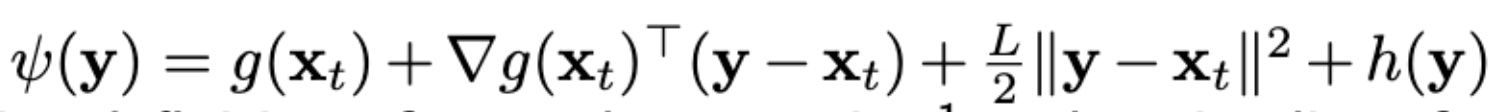### Exercice 25 - strong convexity

Hi,

I think I am missing something for solving exercise 25. How do we know that $$\psi(y)$$ is strongly convex?

Top comment

Hello,$$\psi(y)$$ is a sum of a constant function of $$y$$ (convex), a linear function (convex), a quadratic function (strongly convex) and a convex function (by Theorem 3.14 assumption). Hence, you can show that it is strongly convex. There is a little stretch from the course as you saw the definition of strong convexity when $$f$$ is differentiable but here we would need a definition of strong convexity with sub-gradients as $$h$$ is not necessarily differentiable.

I hope this clarify the solution.
JB

Are you referring to Exercise 25 of the lecture notes? I don't see a $$\psi(y)$$ there?

Top comment

Hello,$$\psi(y)$$ is a sum of a constant function of $$y$$ (convex), a linear function (convex), a quadratic function (strongly convex) and a convex function (by Theorem 3.14 assumption). Hence, you can show that it is strongly convex. There is a little stretch from the course as you saw the definition of strong convexity when $$f$$ is differentiable but here we would need a definition of strong convexity with sub-gradients as $$h$$ is not necessarily differentiable.

I hope this clarify the solution.
JB